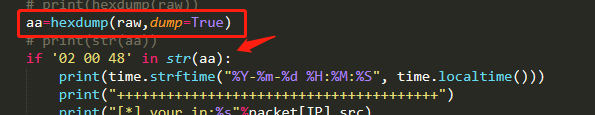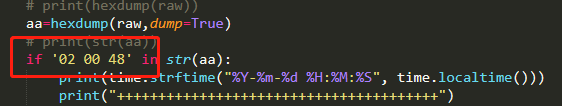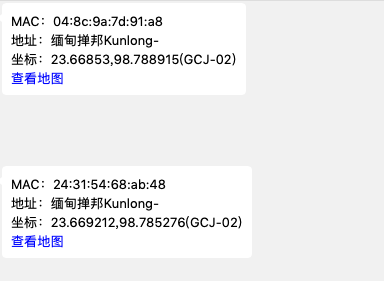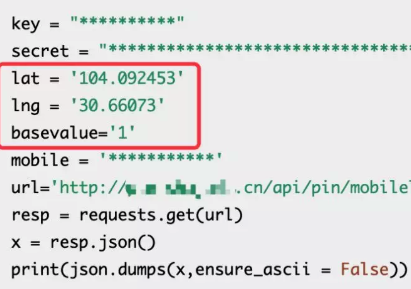# 1.qq抓取会话ip

## 1.2 程序思路## 1.3 程序编写

Github地址：

``````#coding=utf-8

from scapy.all import *

import time

def packet_callback(packet):

try:

raw=packet[Raw]

# print(hexdump(raw))

aa=hexdump(raw,dump=True)

# print(str(aa))

if '02 00 48' in str(aa):

print(time.strftime("%Y-%m-%d %H:%M:%S", time.localtime()))

print("+++++++++++++++++++++++++++++++++++++++")

print("[*] your_ip:%s"%packet[IP].src)

print("[*] your_port:%s"%packet[UDP].sport)

print("--------------------------------")

print("[*] woof_ip:%s"%packet[IP].dst)

print("[*] woof_port:%s"%packet[UDP].dport)

print("+++++++++++++++++++++++++++++++++++++++")

# print(packet.show())

# return

except Exception as e :

pass

# print(e)

# print(packet.show())

# #开启嗅探

sniff(prn=packet_callback,store=0)
``````

## 1.4 扩展思路# 2.被控端进行真实位置获取

## 2.1 前言

netsh wlan show networks mode=bssid

## 2.2 实际操作截图## 2.3 手机号获取经纬度• 通过
• 未通过

0 投票者

xml获取IP更加可靠点